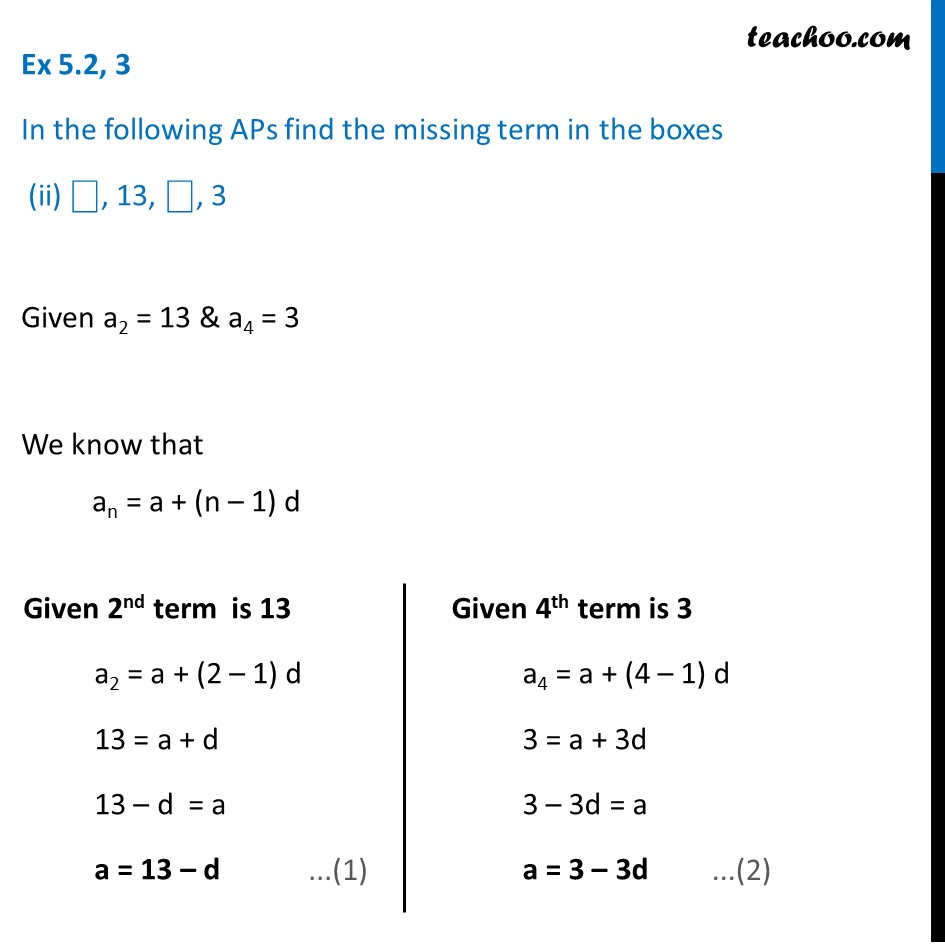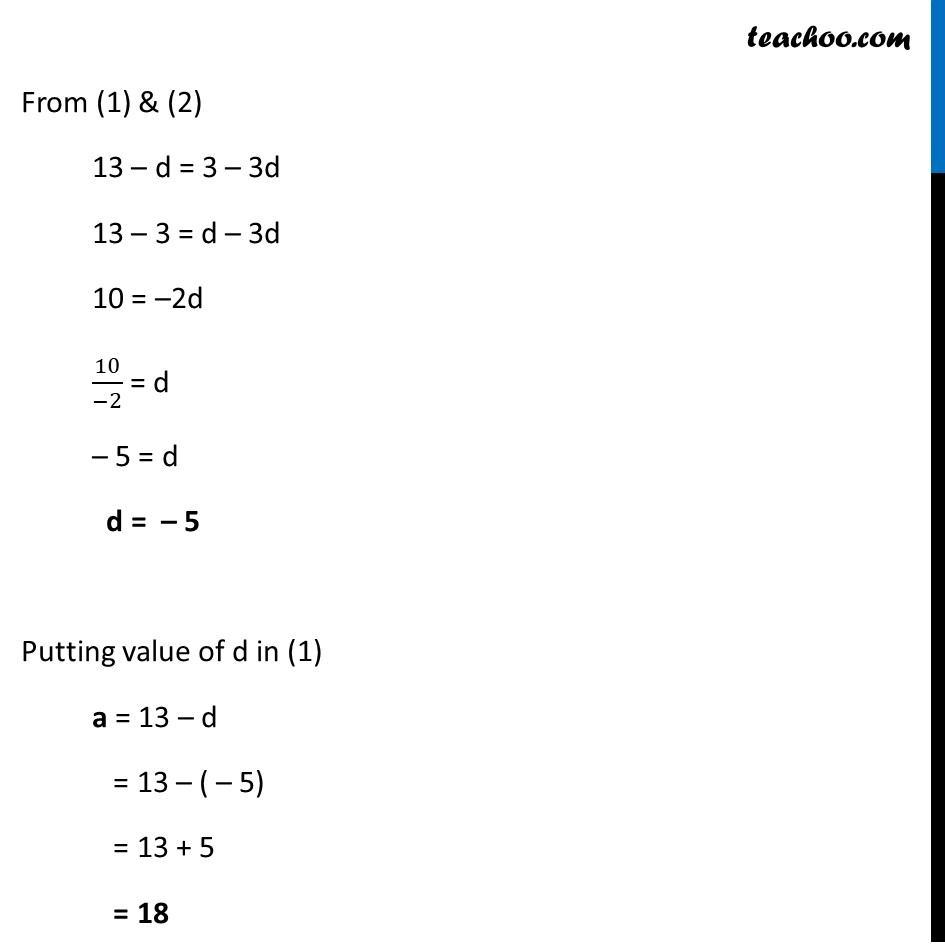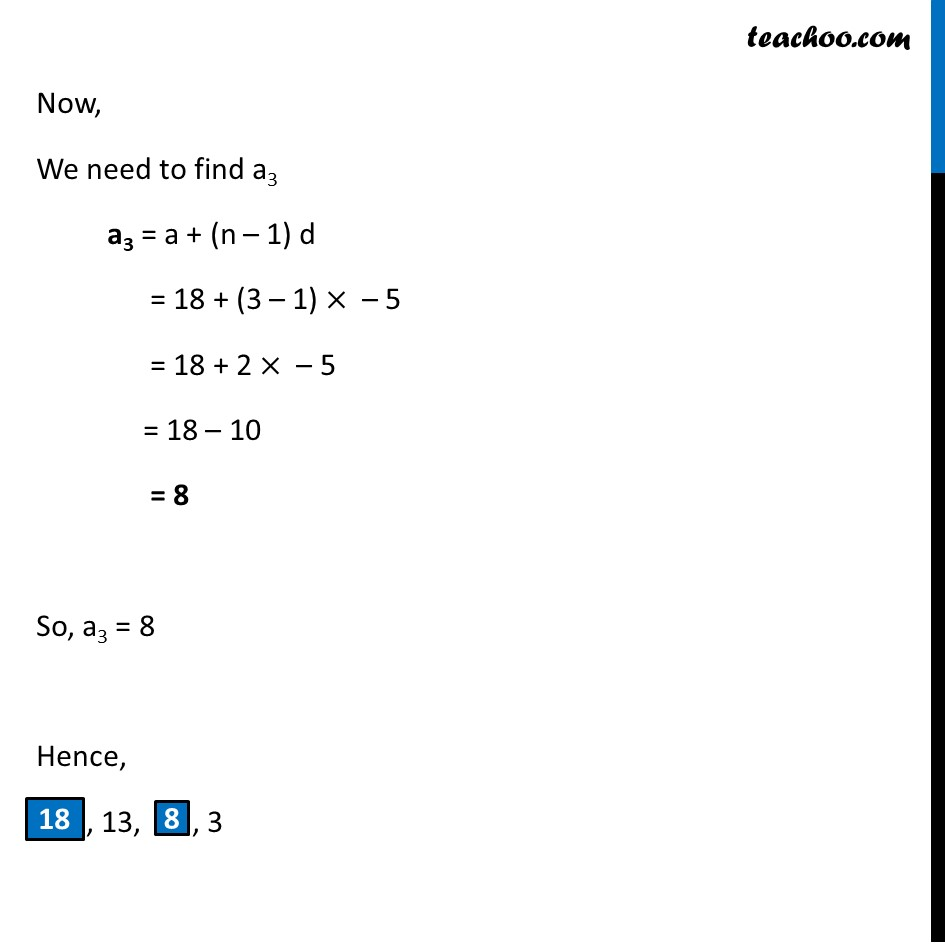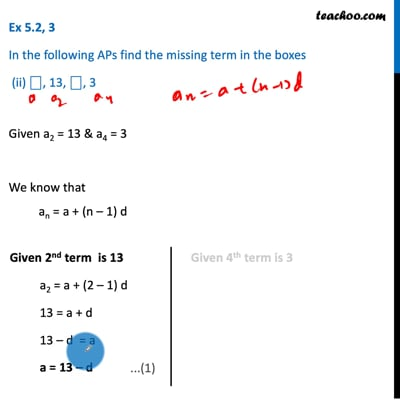Ex 5.2

Chapter 5 Class 10 Arithmetic Progressions
Serial order wiseThis video is only available for Teachoo black users

Maths Crash Course - Live lectures + all videos + Real time Doubt solving!

### Transcript

Ex 5.2, 3 In the following APs find the missing term in the boxes (ii) ⎕, 13, ⎕, 3 Given a2 = 13 & a4 = 3 We know that an = a + (n – 1) d Given 2nd term is 13 a2 = a + (2 – 1) d 13 = a + d 13 – d = a a = 13 – d Given 4th term is 3 a4 = a + (4 – 1) d 3 = a + 3d 3 – 3d = a a = 3 – 3d From (1) & (2) 13 – d = 3 – 3d 13 – 3 = d – 3d 10 = –2d 10/(−2) = d – 5 = d d = – 5 Putting value of d in (1) a = 13 – d = 13 – ( – 5) = 13 + 5 = 18 Now, We need to find a3 a3 = a + (n – 1) d = 18 + (3 – 1) × – 5 = 18 + 2 × – 5 = 18 – 10 = 8 So, a3 = 8 Hence, , 13, , 3